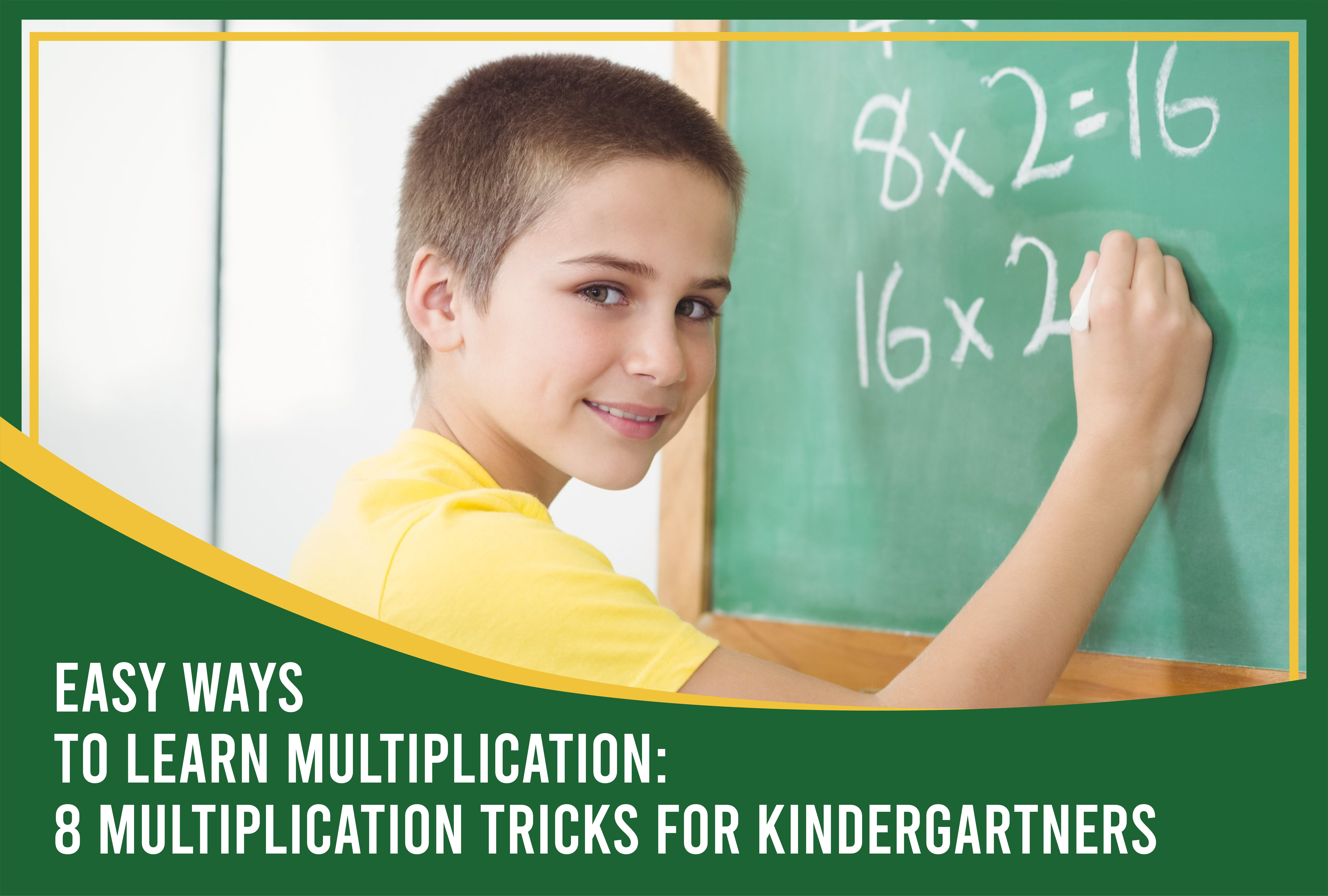Submitted by sam on Wed, 07/06/2022 - 13:07

# Easy Ways to Learn Multiplication: 8 Multiplication Tricks for Kindergartners

Multiplication is a vital part of a child's mathematical education. It lays the foundation for understanding more complex concepts such as algebra and geometry. It also helps children develop problem-solving skills. While it's never too early for kids to start learning multiplication, the leap from learning addition and subtraction to something more complex like multiplication can be daunting and intimidating for some kids.

The good news is that there are easy ways to learn multiplication and help your little one become proficient in this essential math skill. Here are eight multiplication tricks for kindergarteners that will make learning multiplication fun and easy.

One of the simplest ways to help your child learn multiplication is using their fingers. Teachers often use this method in the early stages of teaching multiplication. To use this method, have your child hold up a certain number of fingers based on the number they are multiplying. For example, if they are multiplying by three, they would hold up three fingers. They would then count the total number of fingers held up by both hands to get the answer. So, in this case, the answer would be nine (3 x 3).

### Count by 2s, 3s, 4s, and so on

Another easy way to help your child learn multiplication is by counting by certain numbers. Start with small numbers like two and three. For example, if your child is multiplying by two, have them count each number out loud, starting at zero until they reach the number they are multiplying by (in this case, 10). So they would say "0, 2, 4, 6, 8, 10". Once they get the hang of it, try larger numbers like four and five.

### Use a multiplication chart

A multiplication chart is a great tool to help kids learn and memorize multiplication tables. It's basically a reference chart that shows the product of all the numbers from 1 to 10 (or 1 to 12). You can find multiplication charts online or make your own. Hang it up on the fridge or somewhere where your child can see it and refer to it often.

### Use manipulatives

Kids learn best when they are actively engaged in the learning process. That's why manipulatives are such an excellent tool for teaching multiplication. Manipulatives are objects that children can touch, move, and manipulate to help them understand a concept.

There are many different types of manipulatives that can be used for multiplication. These include unifix cubes, dot cards, number lines, and 100s chart. You can find these manipulatives online or at your local educational store.

### Play multiplication games

Learning doesn't have to be all work and no play. There are many fun and educational games that can help kids learn multiplication. Multiplication war is a popular game played with a deck of cards. To play, each player gets half of the deck and arranges their cards in numerical order from smallest to largest. The first player lays down any card from their hand and calls out its multiplication equation (for example, "4 x 7"). The second player then lays down a card with the same answer and calls out the following equation ("7 x 4"). The first player to lay down all their cards wins the game.

One way to help kids understand multiplication is by relating it to addition. Anchor the concept that multiplication is simply repeated addition. For example, if your child is multiplying 7 x 3, you can have them add 7 + 7 + 7 instead. This will help them see that the answer is the same as if they had added three groups of seven (21).

### Visualize with arrays

An array is a visual representation of data or information using rows and columns. You can use arrays to help kids visualize multiplication equations. For example, the equation 4 x 5 can be represented as an array with four rows and five columns. Each row would represent one group of four, and each column would represent one number multiplied by four (4, 8, 12, 16, 20).

### Keep practicing

Another excellent way to master multiplication is by practicing often. This will help kids memorize the multiplication tables and become more confident in their skills. A great way to practice is by using flashcards. You can make your own flashcards or find them online or at your local educational store. Another option is to use an online multiplication game or app. There are many different games and apps available that are both educational and fun.

Learning how to multiply is a fundamental math skill that will benefit kids throughout their schooling and beyond. With these eight easy tips, your child will be multiplying like a pro in no time!

At Mrs. Myers' Learning Lab, we take pride in our ‘Nifty Numbers’ Math Program encompasses fun, interactive techniques through our small-group classes. Whether it be adding and subtracting, vocabulary, mastering multiplication and division, or even dealing with fractions and decimals, we are confident that we have a math program built for every child’s needs!